Science, Maths & Technology

### Become an OU student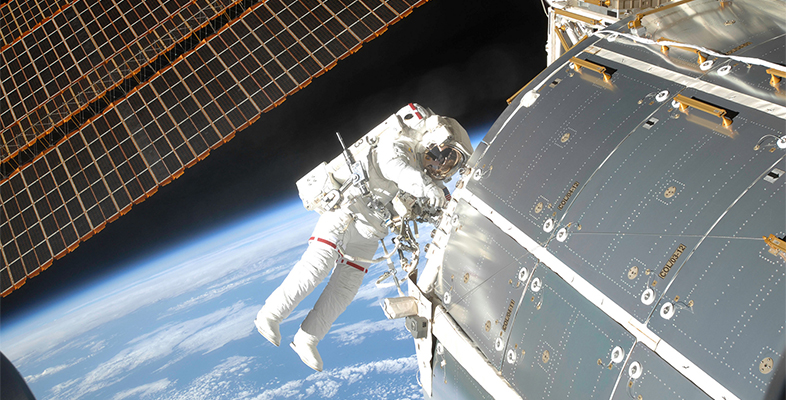Microgravity: living on the International Space Station

Start this free course now. Just create an account and sign in. Enrol and complete the course for a free statement of participation or digital badge if available.

# 4 Reducing the effects of ageing in a microgravity environment

You’ve seen how living in a microgravity environment affects the blood pressure, heart and vision of an astronaut. But how does this environment affect the other senses? Watch Video 2 to see.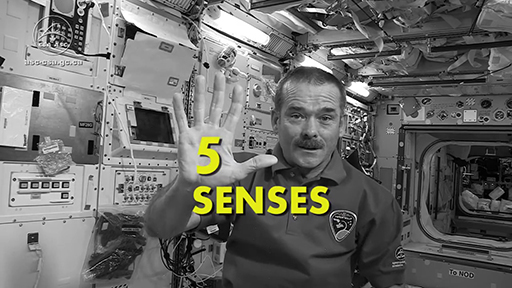Video 2 Hearing in space.
Interactive feature not available in single page view (see it in standard view).

How else does living in a microgravity environment affect the health of an astronaut? Bones and muscles are then weakened further by the reduced effects of Earth’s gravity in a microgravity environment. However, the ‘flip side’ is that prolonged stays in microgravity environments also affect astronauts’ balance, posture and coordination.

Can hormones, drugs and surgical intervention prevent bone loss or encourage bone formation? In the 1940s, Russian scientists developed a surgical technique for promoting bone growth. They found that inserting screws into the bones and gradually forcing the bones apart promotes bone growth (Figure 5).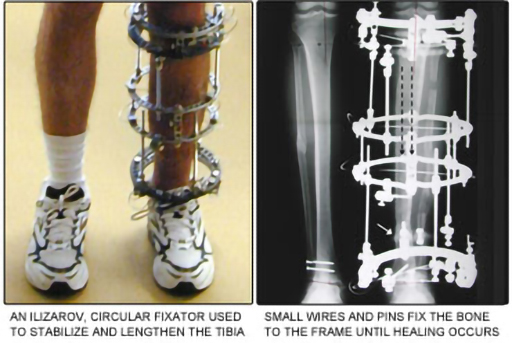Figure 5 External limb-lengthening surgery.

Such a procedure is a bit extreme for astronauts on board the ISS though! Instead, astronauts need to follow a strict physical regime, devoting at least two hours per day to excercise.

What kind of exercises would help to reduce bone and muscle loss? Due to the effects of microgravity, some activities are much easier on the ISS than on Earth: for example, weightlifting. This means that exercise equipment has to be designed specifically to be used in space. You can discover more on this website: www.nasa.gov/ audience/ foreducators/ stem-on-station/ ditl_exercising [Tip: hold Ctrl and click a link to open it in a new tab. (Hide tip)]

Figure 6 shows NASA astronaut Karen Nyberg using the rather exotically named Advanced Resistive Exercise Device (ARED) (weightlifting equipment) on board the ISS. How do you think this has been adapted for use in space?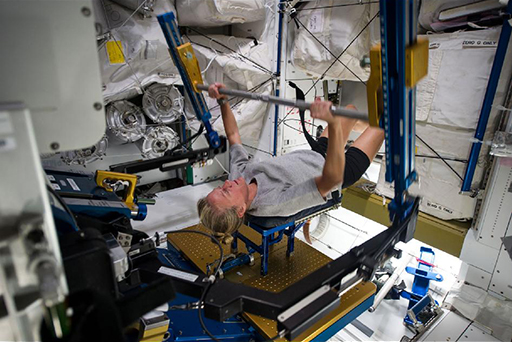Figure 6 Astronaut Karen Nyberg exercising on the ISS in 2015.

Weightlifting is not the only exercise that astronauts can do. They can even run marathons on the ISS. The main adaptation for space use is a harness which attaches the runner to the treadmill (see Figure 7). Here, in 2007, NASA astronaut Sunita Williams completed the first marathon in orbit around the Earth. She ran as an entrant in the Boston Marathon in a respectable time of 4 hours and 24 minutes.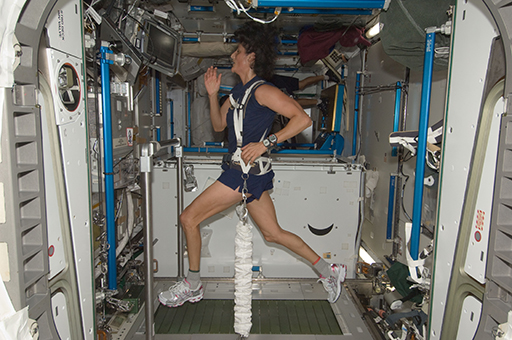Figure 7 NASA astronaut Sunita Williams running on a treadmill on the ISS in 2007.

Now complete Activity 4 which focuses on marathon distances, times and speeds.

Note: some values will need to be rounded to the appropriate number of significant figures. The ‘trick’ here is to look carefully at the number given by your calculation, for example 123 552. If you needed to round this to 2 significant figures, look at the second digit in this number. Here it is 2. The digit immediately after this is 3. As this digit is 4 or less, you leave it alone. If it is between 5 and 9, you round up the previous digit. In the case of 123 552, to 2 significant figures, this would be rounded down to 120 000.

If the value was 7777.77, to 2 significant figures and because the third digit is 7, it would be rounded up to 7800.

## Activity 4 Running a marathon

Timing: Allow approximately 15 minutes

Answer the following questions by choosing the one correct option for each.

1. How far is 42 kilometres in metres?

(Hint: multiply 42 by 1000 to obtain the correct answer in metres.)

a.

42 metres

b.

420 metres

c.

4200 metres

d.

42 000 metres

e.

420 000 metres

2. How long is 4 hours and 24 minutes in seconds?

(Hint: first, change 4 hours and 24 minutes into minutes. Multiply 4 by 60 and then add 24. Then multiply your answer by 60 to obtain the correct answer in seconds.)

a.

26.4 seconds

b.

264 seconds

c.

1584 seconds

d.

2640 seconds

e.

15 840 seconds

3. As the ISS travels at 28 000 km/h, how fast is this in terms of m/s?

(Hint: first, convert 28 000 km to m by multiplying by 1000. Then divide your answer by 3600 to convert hours to seconds. Then round your final answer to 2 significant figures.)

a.

0.78 m/s

b.

7.8 m/s

c.

78 m/s

d.

7800 m/s

e.

780 000 m/s

4. Using the correct value of the speed of the ISS in m/s from Question 3, and the time in seconds in Question 2, how far in km did Sunita and the ISS travel in orbit around the Earth while she was running the marathon?

(Hint: distance is speed times time. Multiply the value from Question 3 by the value in Question 2. Then divide by 1000 to change from m to km. Then round your answer to 2 significant figures.)

a.

0.12 km

b.

12 km

c.

120 km

d.

1200 km

e.

120 000 km